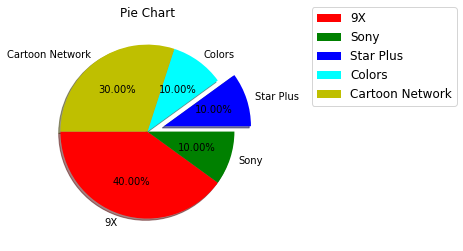Breaking News# Pie Plot using Matplotlib

4. Pie Plot

### Plot a Pie Chart using Matplotlib¶

Pie charts represent data broken down into categories/labels. They’re an intuitive and simple way to visualize proportional data – such as percentages.

To plot a pie chart in Matplotlib, we can call the pie() function of the PyPlot or Axes instance. The only mandatory argument is the data we’d like to plot, such as a feature from a dataset:

In :
import matplotlib.pyplot as plt
x = [15, 25, 25, 30, 5]
plt.pie(x)
plt.show()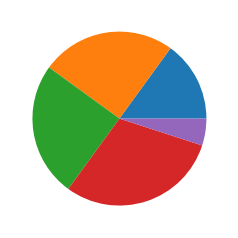This generates a rather simple, but plain, Pie Chart with each value being assigned to a proportionally large slice of the pie:

In :
import matplotlib.pyplot as plt
x = [15, 25, 25, 30, 5]
plt.pie(x,wedgeprops={'edgecolor': 'black'})
plt.show()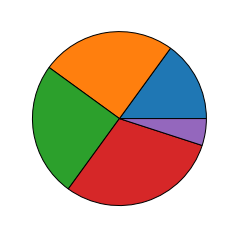### Let’s add some labels, so that it’s easier to distinguish what’s what here:¶

In :
import matplotlib.pyplot as plt
x = [15, 25, 25, 30, 5]
labels = ['9X','Sony','Star Plus','Colors','Cartoon Network']
plt.pie(x,labels = labels)
plt.title('Pie Chart')
plt.show()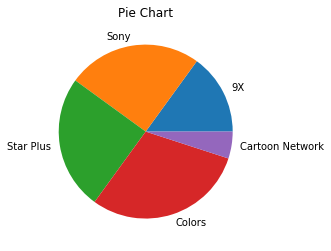### Change Pie Chart Colors¶

To change the colors of a Pie Chart in Matplotlib, we’ll need to supply an array of colors to the colors argument, while plotting it:

In :
import matplotlib.pyplot as plt
x = [15, 25, 25, 30, 5]
labels = ['9X','Sony','Star Plus','Colors','Cartoon Network']
cols=['red','green','b','cyan','y']
plt.pie(x,labels = labels,colors=cols)
plt.title('Pie Chart')
plt.show()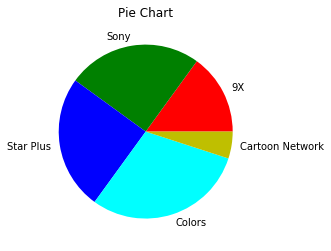### Show Percentages on Slices¶

To add numerical percentages to each slice, we use the autopct argument. It automatically sets the percentages in each wedge/slice, and accepts the standard Python string formatting notation:

In :
import matplotlib.pyplot as plt
plt.title("Pie Chart")
slices=[40,10,10,10,30]
channels=['9X','Sony','Star Plus','Colors','Cartoon Network']
cols=['red','green','b','cyan','y']
plt.pie(slices,labels=channels,colors=cols,autopct='%.2f%%')
plt.show()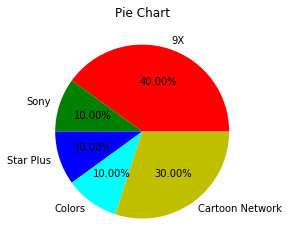### Explode/Highlight Wedges¶

Setting this value to 1 would offset it by a lot, relative to the chart, so usually, you’ll explode wedges by 0.1, 0.2, 0.3, and similar values. You can explode as many of them as you’d like, with different values to highlight different categories.

In :
import matplotlib.pyplot as plt
plt.title("Pie Chart")
slices=[40,10,10,10,30]
channels=['9X','Sony','Star Plus','Colors','Cartoon Network']
cols=['red','green','b','cyan','y']
plt.pie(slices,labels=channels,colors=cols,autopct='%.2f%%',explode=(0,0,0.2,0,0))
plt.show()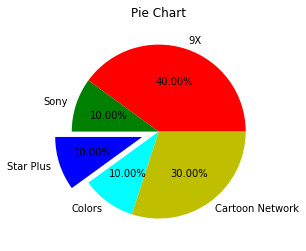To add a shadow to a Matplotlib pie chart, all you have to do is set the shadow argument to True:

In :
import matplotlib.pyplot as plt
plt.title("Pie Chart")
slices=[40,10,10,10,30]
channels=['9X','Sony','Star Plus','Colors','Cartoon Network']
cols=['red','green','b','cyan','y']
plt.show()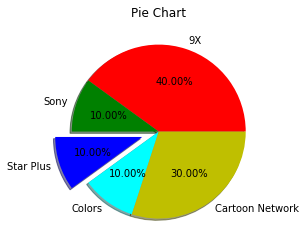### Rotating Pie Chart¶

Finally, you can also rotate the chart, by setting the starting angle. So far, it starts on 0 degrees (right-hand), and populated wedges counter-clockwise. By setting the startangle argument to a number between 0..360, you can make a full circle:

In :
import matplotlib.pyplot as plt
plt.title("Pie Chart")
slices=[40,10,10,10,30]
channels=['9X','Sony','Star Plus','Colors','Cartoon Network']
cols=['red','green','b','cyan','y']
plt.show()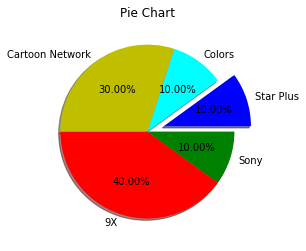### Final Pie Chart¶

In :
import matplotlib.pyplot as plt
plt.title("Pie Chart")
slices=[40,10,10,10,30]
channels=['9X','Sony','Star Plus','Colors','Cartoon Network']
cols=['red','green','b','cyan','y']
plt.legend(loc="upper right",fontsize=12,bbox_to_anchor=(1.95,1.1))
plt.savefig('plot.png')
plt.show()